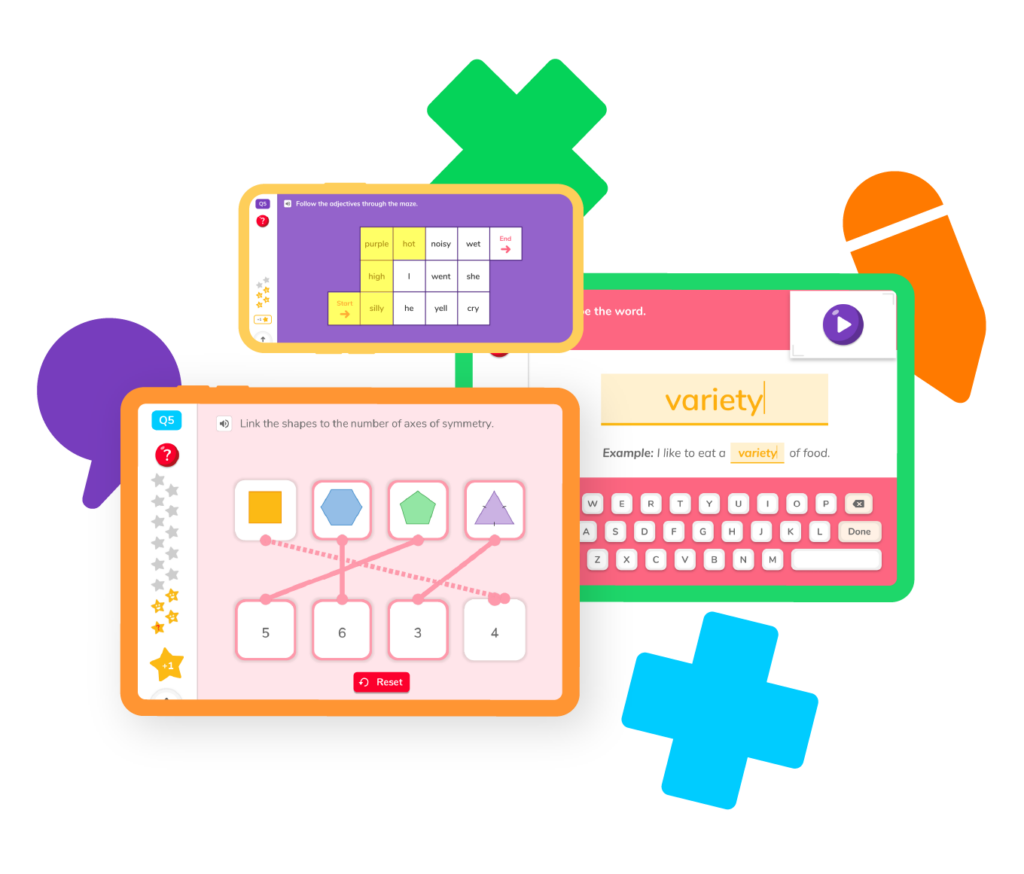# Learning Division

Division can be one of the hardest math skills to master, but it’s super important as students advance to higher-level mathematics. Whether they’re trying to discover how to divvy up pieces of pizza to give to their friends or solving complex problems in high school classes like precalculus and calculus, elementary schoolers will want to start practicing division skills now to make sure they’re ready – both for the classroom and the real world!## Division resourcesLike almost all mathematical skills, division starts out simple and becomes more complex as you move through elementary school. Let’s take a look at different division skills to make sure we’re prepared at every stage of learning.

## Basic division

Start by breaking products down into quotients.

## Long division

Master this tough skill and the rest is easy-peasy.

## Remainders

Practice finding remainders both in basic and long division.

## Dividing fractions

Use other math skills like multiplication to divide fractions.

## Division word problems

Learn how to solve word problems that require division.

## Terms to know

Memorize important division terms like divisor, dividend and more.

## Basic division

Start by breaking products down into quotients.

## Long division

Master this tough skill and the rest is easy-peasy.

## Remainders

Practice finding remainders both in basic and long division.

## Dividing fractions

Use other math skills like multiplication to divide fractions.

## Division word problems

Learn how to solve word problems that require division.

## Terms to know

Memorize important division terms like divisor, dividend and more.Division is a bit more complex than basic mathematical skills like addition or subtraction. As the inverse operation of multiplication, it may seem easy at first, but the more you mess with uneven numbers, fractions, and decimals, the more complicated it becomes. Let’s take a look at some common questions about division.

Division is important because it helps us split things into equal parts. This can help us share a portion of something equally or help us divide our time during the day so we can accomplish the tasks ahead of us. You’ll use division almost every day as you get older!

It might sound simple, but the remainder is what is left over after a division problem has been completed. So, for example, it’s impossible to divide 16 by 5 and get a whole number. But, the number 15 is divisible by 5. If we divide 16 by 5, we get the answer 3 with a remainder 1.

A quotient is the answer you get when you divide one number by another. With addition, we call this answer a “sum,” with subtraction, we call it the “difference,” and with multiplication, we call it the “product.”

Long division is the process we use to divide large numbers, with multiple digits, into smaller numbers. It can take a bit of getting used to, and there are several steps involved in the process, but don’t worry – DoodleMath has the perfect guide to help you perfect your long division skills!# Are you a parent, teacher or student?

Are you a parent or teacher?

## Hi there!

Book a chat with our team